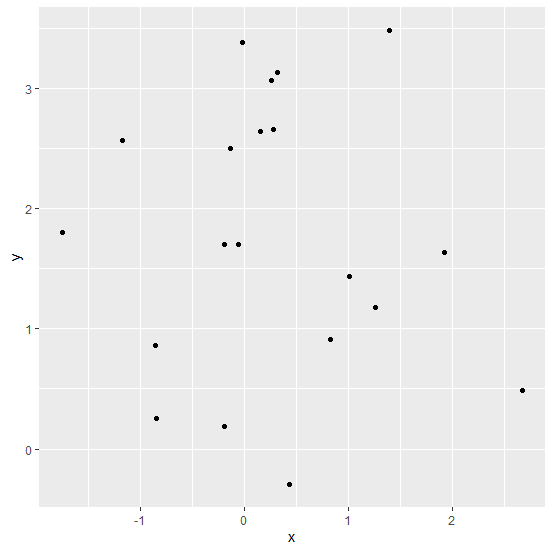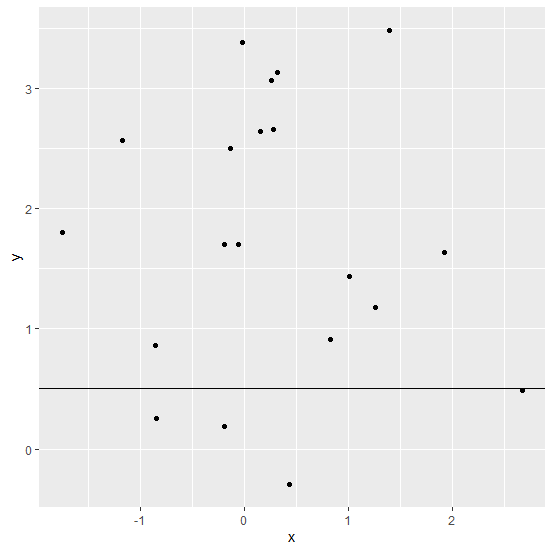# How to add a horizontal line to the plot created by ggplot2 in R?

When we create a plot, it shows the values passed by the function for creating the plot but we might want to display some other values to provide some information through the plot and that information could be a threshold value as a horizontal line or we can also call it a cut off value. This can be done by using geom_hline function of ggplot2 package.

## Example

Consider the below data frame −

> x<-rnorm(20)
> y<-rnorm(20,1.5)
> df<-data.frame(x,y)
> df

## Output

      x       y
1 0.27810573 2.6545571
2 1.39185082 3.4845292
3 -0.19068920 1.7043852
4 1.00791317 1.4324814
5 -1.74964913 1.7996093
6 -0.13123079 2.5004350
7 0.15729145 2.6425085
8 0.42815918 -0.2970325
9 -0.84252471 0.2579510
10 0.25413824 3.0670546
11 -0.05608811 1.6974104
12 -0.85671276 0.8638574
13 -1.17183043 2.5650640
14 2.67224782 0.4832468
15 -0.01763065 3.3835275
16 1.26122484 1.1755709
17 1.91652453 1.6351443
18 0.82211772 0.9123337
19 -0.19153555 0.1831160
20 0.31878745 3.1280550

Creating a scatterplot between x and y −

> library(ggplot2)
> ggplot(df,aes(x,y))+geom_point()

## OutputAdding a horizontal line in the above plot −

> ggplot(df,aes(x,y))+geom_point()+geom_hline(yintercept=0.5)

## Output